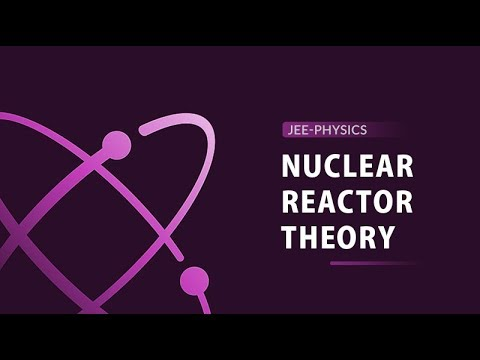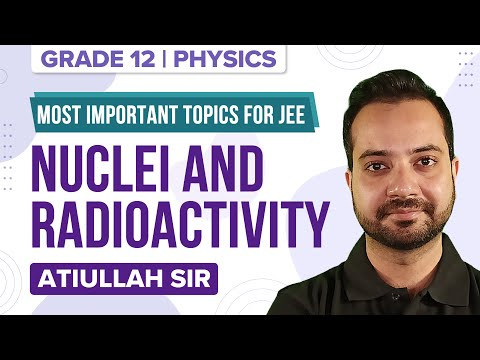Jet Set Go! All about Aeroplanes Jet Set Go! All about Aeroplanes

# Nuclear Reactions Questions

The Nuclear reactions lead to the transformation of the participating nuclei forming new nuclides and energy. The nuclear fusion of lighter elements is responsible for the generation of heat and light energies in the stars and sun.

 Definition: It is the process of formation of a new nuclide by the interaction of two nuclei or by the interaction of a nucleus with high energy radiations.

## Nuclear Reactions Chemistry Questions with Solutions

Q1: The atomic mass unit is

1. 1/12th the mass of 1 mole of carbon atoms
2. 1/12th the mass of 1 mole of oxygen atoms
3. 1/12th the mass of an oxygen atom
4. 1/12th the mass of a carbon atom

Explanation: The atomic mass unit is equal to 1/12th the mass of a carbon atom. It is denoted by the unit “u” and is equal to 1.66 x 10-27 kg.

Q2. The spectroscopic measurement of the atomic masses reveals that

1. All atoms have the same core
2. All atoms have a proton and a neutron
3. There exists different atoms of the same element
4. Carbon is the smallest unit for measurement of mass

Explanation: The spectroscopic measurement of the atomic masses reveals that there exists different atoms of the same element. While all of these atoms have the same chemical properties, they have different mass. Such atoms of an element that differ in mass are called Isotopes of that element.

Q3. Emission of which one of the following does not change the atomic number and mass number of the element?

1. An alpha particle
3. Beta particles
4. Positron

Explanation: The gamma rays leave the atomic number and mass numbers unaltered when they leave. Gamma rays in turn, lower down the energy of the nucleus from a high energy state to a lower energy state. This happens by the release of some electromagnetic radiation by the nucleus.

Q4. Which one of the given radiations is the least penetrating?

1. Alpha rays
2. Beta rays
3. Gamma rays
4. X-rays

Explanation: Alpha particles have the highest mass out of all of the ionizing radiations. The high mass makes the alpha particles most ionizing. However due to the large mass, the penetration of the alpha particles is the least (only a few cm through the air) among other ionizing radiations.

Q5. Predict the age of a bottle of wine if the measured tritium (3H) content for the old bottle is 25% of that of the new wine? The half life of 3H is 12.5 years.

1. 50 years
2. 25 years
3. 100 years
4. 37.5 years

Explanation: To get reduced to 25% of its original amount, tritium would undergo two half-lives.

$$\begin{array}{l}^{3}H\ \xrightarrow[Half-life]{First}\ 50\ percent\ ^{3}H\\50\ percent\ ^{3}H\ \xrightarrow[Half-life]{Second}\ 25\ percent\ ^{3}H\end{array}$$

Hence, first half-life + second half-life = 12.5 + 12.5 = 25 years

Q6. Atomic mass is the average mass. Explain.

Answer: In the naturally existing element, some atoms of its isotopes are also found. Different elements have different abundances of these atoms. Hence, to determine the mass of an atom of an element, an average of the masses of these isotopes as per their natural abundance is taken. Hence, the atomic mass is an average mass of the atom.

Q7. What are the four main kinds of nuclear reactions?

Answer: The four different types of nuclear reactions are:

1. Nuclear fusion: In this reaction, two lighter nuclei combine together to form a heavier nucleus. This reaction releases energy as the mass of the new nucleus formed is less than the combined mass of the two initial nuclei. Hence, the leftover mass is converted into energy.
2. Nuclear fission: In this reaction a heavy nucleus gets divided to form two new nuclei of approximately the same masses. This reaction releases a large amount of energy.
3. Nuclear / Radioactive decay: In this reaction, an unstable nucleus keeps on losing its energy in the form of radiations.
4. Nuclear Transmutation: In this reaction, an element gets converted into some other kind of element. This happens when the number of protons and neutrons present inside the nucleus changes.

Q8. What changes are brought about by the nuclear reactions within an atom?

Answer: Nuclear reactions mainly affect an atom’s nucleus. In most of the cases, after a nuclear reaction, an element is converted into a different element. Hence, the number of protons and neutrons may change during the nuclear reactions.

Q9. Which kind of nuclear reaction emits a high amount of energy?

Answer: Nuclear Fission releases the maximum amount of energy among any other nuclear reactions. The release of energy is accompanied by the loss of neutrons, gamma rays and other sub-nuclear radiations. Fission occurs when a neutron slams into a large atom with a great speed causing the breakup of the larger atom.

Q10. Is there any involvement of electrons in the nuclear reactions?

Answer: Nuclear reactions are entirely based on the nucleus of an atom. All the energy changes take place within the nucleus be it the change in the number of protons and neutrons or the release of energy. Hence, electrons are not involved in any of the nuclear reactions.

Q11. Are all nuclear reactions radioactive?

Answer: Nuclear reactions are often initiated by the bombardment of high energy radiations on a nucleus causing the nucleus to convert into the nucleus of another element. Radioactivity occurs in heavier atoms (above atomic number 83) due to the instability of the heavier nucleus. The nucleus tries to attain stability by relieving some of its nucleons or some energy. Hence, not all nuclear reactions are radioactive in nature.

Q12. Can an ongoing nuclear reaction be stopped?

Answer: No, an ongoing nuclear reaction cannot be stopped until all of its reactants are used up or until all the unstable nuclei undergo a decay.

Q13. Do environmental conditions have any impact on the nuclear reactions?

Answer: The environmental conditions such as temperature and pressure do not have any effect on the nuclear reactions. As for the excitation of a nucleon, the amount of energy required is in the range of 107 K. Hence, the temperature changes from 10s, 10s, 1000s or 10000s K are not sufficient to bring about the excitation of a nucleon. However, if a temperature higher than 107 K could be applied, the nucleus would start undergoing all sorts of nuclear reactions just like in stars.

Q14. Out of the nuclear reactions and chemical reactions, which one emits more energy and why?

Answer: Nuclear reactions include fusion and fission that release huge amounts of energy owing to the very strong force of attraction between the nucleons. However, in chemical reactions, only loosely held electrons are involved in the reaction. This exchange of electrons does not release even comparable amounts of energy to that of the nuclear reactions. The energy released from nuclear reactions is million times more than those released from the chemical reactions.

Q15. What is a mass defect?

Answer: It is the difference between the actual mass of an atom and the mass calculated by calculating the number of protons and neutrons present in the nucleus. The actual mass is always less than the predicted mass.

## Practise Questions on Nuclear Reactions

Q1. The mass number and charge of a positron are

1. 0 and +1
2. 0 and 2+
3. 1 and 1+
4. 1 and 2+

Q2. How is Nuclear power generated?

Q3. What is an alpha-emitter?

Q4. Which nuclear reaction takes place in the sun?

Q5. What are gamma rays? How are they produced?

Click the PDF to check the answers for Practice Questions.

## Theory of Nuclear Reactor